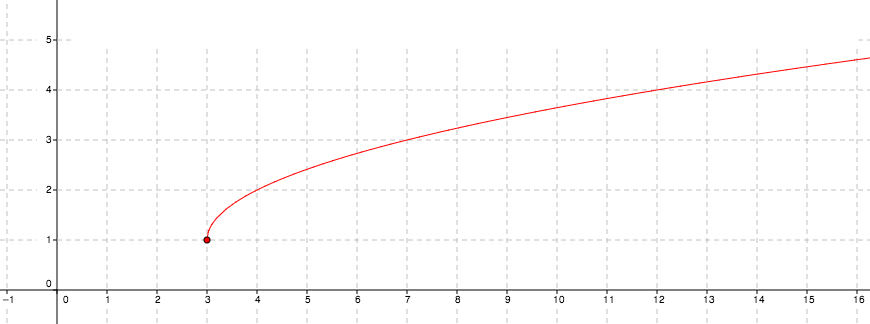### Home > AC > Chapter 12 > Lesson 12.3.1 > Problem12-56

12-56.

Graph the function $g\left(x\right)=\sqrt{x-3}+1$ on graph paper. Describe its domain and range.The domain is the set of values $x$ can be.

The range is the set of values $y$ can be.

The graph starts at $\left(3,1\right)$.
Domain: $x\ge3$
Range: $y ≥ 1$